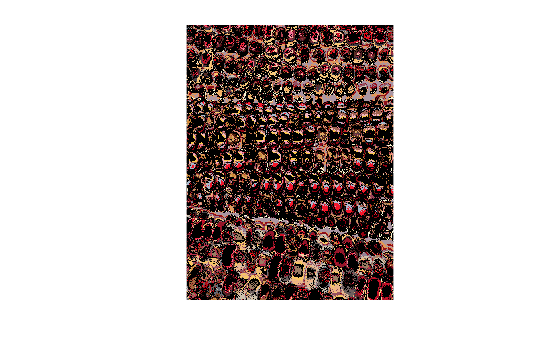# cmpermute

Rearrange colors in colormap

## Syntax

``[Y,newmap] = cmpermute(X,map)``
``[Y,newmap] = cmpermute(X,map,index)``

## Description

example

````[Y,newmap] = cmpermute(X,map)` randomly reorders the colors in colormap `map` to produce a new colormap, `newmap`. The `cmpermute` function also modifies the values in indexed image `X` to maintain correspondence between the indices and the colormap, and returns the result in `Y`. The image `Y` and associated colormap, `newmap`, produce the same image as `X` and `map`.```
````[Y,newmap] = cmpermute(X,map,index)` uses an ordering matrix (such as the second output of `sort`) to define the order of colors in the new colormap.```

## Examples

collapse all

Read the first image, `X`, and its associated colormap, `map`, from the sample indexed image file, `corn.tif`. Display the image.

```[X,map] = imread('corn.tif'); imshow(X,map)```Randomly reorder the colormap to get a new colormap, `newmap`. Display image `X` with the new colormap.

```[Y,newmap] = cmpermute(X,map); colormap(newmap)```## Input Arguments

collapse all

Indexed image, specified as an m-by-n matrix of integers.

Data Types: `double` | `uint8`

Colormap associated with indexed image `X`, specified as a c-by-3 matrix with values in the range [0, 1]. Each row of `map` is a three-element RGB triplet that specifies the red, green, and blue components of a single color of the colormap.

Data Types: `double`

Sort index, specified as a c-element vector of positive integers.

Data Types: `double`

## Output Arguments

collapse all

Indexed image, returned as an m-by-n matrix of integers. `Y` has the same data type as input indexed image `X`.

Data Types: `double` | `uint8`

Colormap with reduced colors associated with the output indexed image `Y`, returned as a c-by-3 matrix with values in the range [0, 1]. Each row of `newmap` is a three-element RGB triplet that specifies the red, green, and blue components of a single color of the colormap.

Data Types: `double`